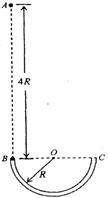﻿ 

# 题型：功、功率和功能关系的计算题

### 1. 几种力做功的特点

#### 2. 摩擦力做功的特点

* ①单个摩擦力(包括静摩擦力和滑动摩擦力)可以做正功、也可以做负功,还可以不做功 * ②相互作用的一对静摩擦力做功的代数和为0         在静摩擦力做功的过程中,只有机械能的转移,没有机械能转化为其他形式的能:相互作用的一对滑动摩擦力做功的代数和一定不为0,且总为负值;在一对滑动摩擦力做功的过程中,不仅有相互摩擦的物体间机械能的转移,还有部分机械能转化为内能,转化为内能的量等于机械能的减少量,等于滑动摩擦力与相对运动位移的乘积。 * ③摩擦生热是指滑动摩擦生热,静摩擦不会生热

#### 2. 几个重要的功能关系

1. 重力的功等于重力势能的变化,即${W}_{f}=-{\mathrm{△E}}_{\mathrm{pG}}$ 2. 弹力的功等于弹性势能的变化,即${W}_{弹}=-{\mathrm{△E}}_{\mathrm{p弹}}$ 3. 合力的功等于动能的的变化,即$W={\mathrm{△E}}_{k}$ 4. 重力(或系统内弹簧弹力)之外的其他力的功等于机械能的变化,即${W}_{\mathrm{其他}}=\mathrm{△E}$ 5. 一对滑动摩擦力做的功等于热量的变化，即$Q={F}_{f}·{x}_{\mathrm{相对}}$

1. 相关规律和方法

2. 解题技巧

## 例题 1. 如图所示，光滑绝缘的细圆管弯成半径为R的半圆形，固定在竖直平面内，管口B、C的连线是水平直径．现有一带正电的小球(可视为质点)从B点正上方的A点自由下落，A、B两点间距离为4R．从小球进入管口开始，整个空间中突然加上一个匀强电场，电场力在竖直向上的分力大小与重力大小相等，结果小球从管口C处脱离圆管后，其运动轨迹经过A点．设小球运动过程中带电量没有改变，重力加速度为g，求：(1)小球到达B点的速度大小； (2)小球受到的电场力的大小； (3)小球经过管口C处时对圆管壁的压力．

### 解析:

(1)小球由A自由下落到管口B的过程中机械能守恒，故有：

$\mathrm{mg}·4R=\begin{array}{c}1\\ -\\ 2\end{array}m{{v}_{B}}^{2}$ ${v}_{B}=\sqrt{8\mathrm{gR}}$

（2）设电场力的竖直分力为Fy，水平分力为Fx，则Fy=mg(方向竖直向上)

$x=2R=\begin{array}{c}1\\ -\\ 2\end{array}{a}_{x}{t}^{2}=\begin{array}{c}{F}_{x}\\ ----\\ 2m\end{array}{t}^{2}$

（3）小球经过管口C处时， ${v}_{c}=2\sqrt{\mathrm{gR}}$

### 本题反思：

1. 第一问需要注意运用机械能守恒定律进行求解。

2. 第二问需要抓住

#### 结果小球从管口C处脱离圆管后，其运动轨迹经过A点

3. 注意圆周运动的关系式，有利于解题。